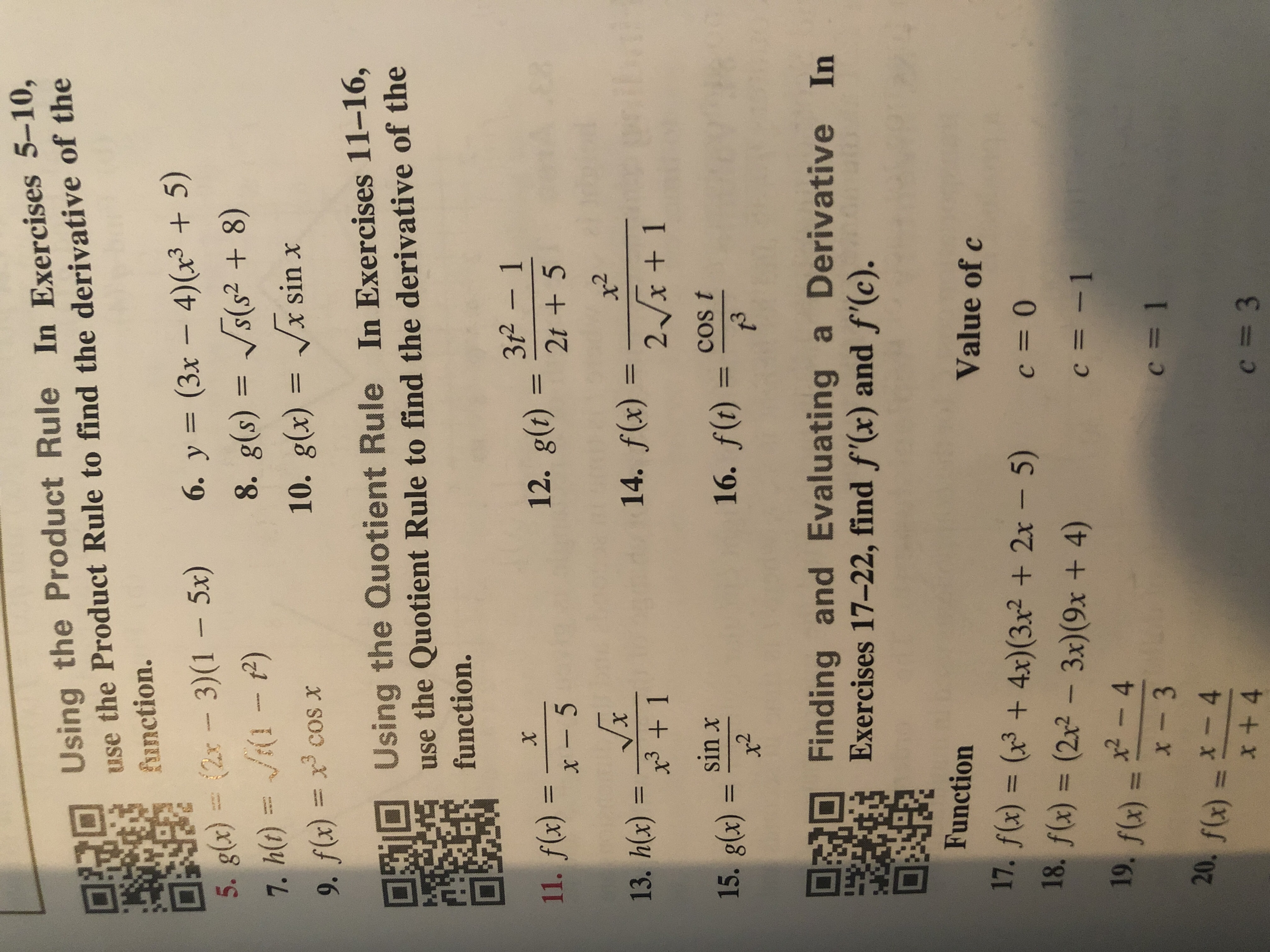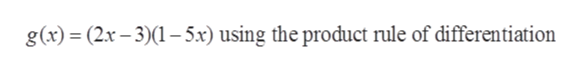Using the Product Rule In Exercises 5-10,use the Product Rule to find the derivative of thefunction.6. y (3x - 4)(x3 +5)8. g(s) s(s2 + 8)10. g(x)= x sin x2x- 3)(1 - 5x)5. g(४) =7. ht)- P)9. f(x)= x cosxUsing the Quotient Rule In Exercises 11-16,use the Quotient Rule to find the derivative of thefunction.3r2 112. g(t)2t +511. f(x)x5x- 514. f(x) 2x+ 113. hx)3 115. glx)Sinx15. g(४) =x2COs t16. f(t)t3sFinding and Evaluating a Derivative InExercises 17-22, find f'(x) and f'(c).FunctionValue of c17. f(x) (3 4x) (3x2 2x-5)18. f(x) = (22- 3x) (9x + 4)c 0wc -119. f(x)2-4x - 3sec = 120. f(x)4c 3x + 4

Question

13help_outlineImage TranscriptioncloseUsing the Product Rule In Exercises 5-10, use the Product Rule to find the derivative of the function. 6. y (3x - 4)(x3 +5) 8. g(s) s(s2 + 8) 10. g(x)= x sin x 2x- 3)(1 - 5x) 5. g(४) = 7. ht) - P) 9. f(x)= x cosx Using the Quotient Rule In Exercises 11-16, use the Quotient Rule to find the derivative of the function. 3r2 1 12. g(t)2t +5 11. f(x)x5 x- 5 14. f(x) 2x+ 1 13. hx)3 1 15. glx)Sinx 15. g(४) = x2 COs t 16. f(t) t3s Finding and Evaluating a Derivative In Exercises 17-22, find f'(x) and f'(c). Function Value of c 17. f(x) (3 4x) (3x2 2x-5) 18. f(x) = (22- 3x) (9x + 4) c 0 w c -1 19. f(x)2-4 x - 3 se c = 1 20. f(x) 4 c 3 x + 4 fullscreen
Step 1

Note: We will answer the question (5) since the exact one wasn’t specified. And it has the multiple questions from (5-10). Please submit a new question specifying the one you’d like to answered.

Step 2

The given function is

Step 3

And it is required to find the d...help_outlineImage Transcriptioncloseg(x) = (2.x-3)(1-5x) using the product rule of differentiation fullscreen

Want to see the full answer?

See Solution

Want to see this answer and more?

Our solutions are written by experts, many with advanced degrees, and available 24/7

See Solution
Tagged in

Derivative